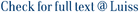We are interested in the convergence of the value of n-stage games as n goes to infinity and the existence of the uniform value in stochastic games with a general set of states and finite sets of actions where the transition is commutative. This means that playing an action profile a1 followed by an action profile a2, leads to the same distribution on states as playing first the action profile a2 and then a1. For example, absorbing games can be reformulated as commutative stochastic games. When there is only one player and the transition function is deterministic, we show that the existence of a uniform value in pure strategies implies the existence of 0-optimal strategies. In the framework of two-player stochastic games, we study a class of games where the set of states is Rm and the transition is deterministic and 1-Lipschitz for the L1 -norm, and prove that these games have a uniform value. A similar proof shows the existence of an equilibrium in the nonzero-sum case. These results remain true if one considers a general model of finite repeated games, where the transition is commutative and the players observe the past actions but not the state.

Commutative stochastic games / Venel, Xavier Mathieu Raymond. - In: MATHEMATICS OF OPERATIONS RESEARCH. - ISSN 0364-765X. - 40:2(2015), pp. 403-428. [10.1287/moor.2014.0676]

### Commutative stochastic games

#### Abstract

We are interested in the convergence of the value of n-stage games as n goes to infinity and the existence of the uniform value in stochastic games with a general set of states and finite sets of actions where the transition is commutative. This means that playing an action profile a1 followed by an action profile a2, leads to the same distribution on states as playing first the action profile a2 and then a1. For example, absorbing games can be reformulated as commutative stochastic games. When there is only one player and the transition function is deterministic, we show that the existence of a uniform value in pure strategies implies the existence of 0-optimal strategies. In the framework of two-player stochastic games, we study a class of games where the set of states is Rm and the transition is deterministic and 1-Lipschitz for the L1 -norm, and prove that these games have a uniform value. A similar proof shows the existence of an equilibrium in the nonzero-sum case. These results remain true if one considers a general model of finite repeated games, where the transition is commutative and the players observe the past actions but not the state.
##### Scheda breve Scheda completa Scheda completa (DC)2015
Markov decision process, stochastic games, uniform value, 0-optimality, repeated games
Commutative stochastic games / Venel, Xavier Mathieu Raymond. - In: MATHEMATICS OF OPERATIONS RESEARCH. - ISSN 0364-765X. - 40:2(2015), pp. 403-428. [10.1287/moor.2014.0676]
File in questo prodotto:
File
Commutative_editor_version.pdf

Solo gestori archivio

Tipologia: Versione dell'editore
Licenza: Tutti i diritti riservati
Dimensione 336.19 kB
Utilizza questo identificativo per citare o creare un link a questo documento: `https://hdl.handle.net/11385/197469`
•7
•7